• 针孔相机模型 相机平面上有两个平面：物理成像平面坐标系和像素平面坐标系 物理成像平面的单位为米，原点在中心 像素平面的单位为像素，原点在边角上 两者之间相差了一个缩放和原点的平移 归一化平面上的投影 ...
针孔相机模型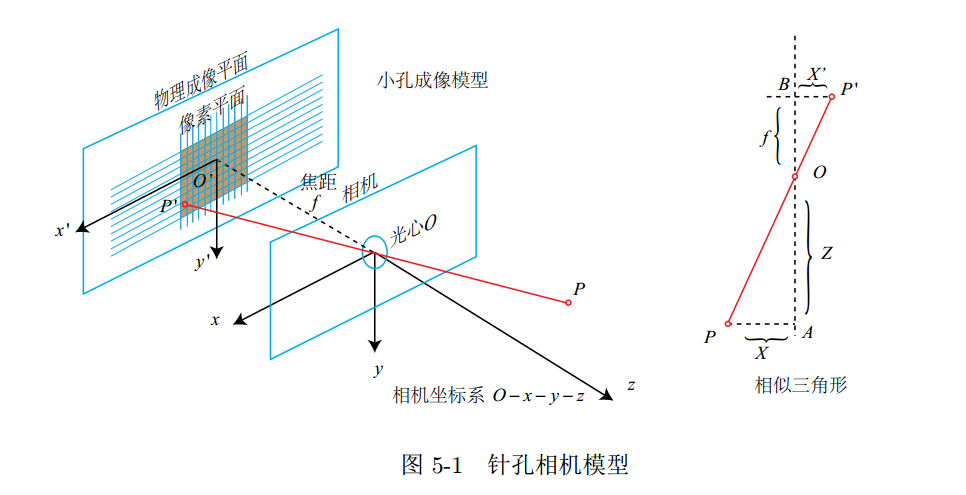相机平面上有两个平面：物理成像平面坐标系和像素平面坐标系

物理成像平面的单位为米，原点在中心 像素平面的单位为像素，原点在边角上 两者之间相差了一个缩放和原点的平移

归一化平面上的投影 指的是在相机坐标系下，点的Z坐标归一化的投影
针孔模型下，相机坐标表下的坐标与像素坐标的映射关系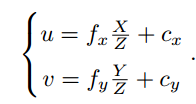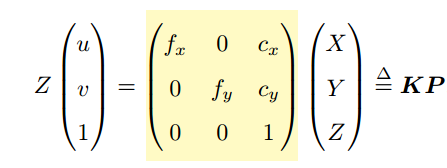在应用中，往往是已知像素坐标，反推相机坐标系下的坐标
                Eigen::Vector3d point;
point = double(d)/depthScale;
point = (u-cx)*point/fx;
point = (v-cy)*point/fy;


双目相机模型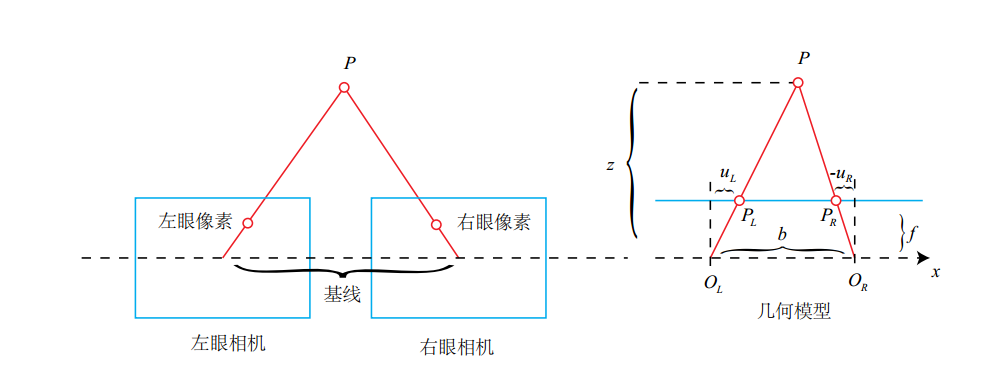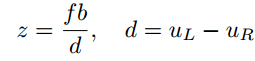深度可以根据双目算出来，关键是同一个像素点在左右图的像素坐标视差的计算是件困难的事情。
展开全文• 1、针孔相机模型、相机畸变、相机标定 2、双目相机模型、RGBD相机原理 2、双目相机与RGBD相机对比 1、单目相机模型 1.1 针孔相机模型 1.2 相机畸变 1.3 相机标定（张正友标定） 1.4 鱼眼相机模型 2、立体相...
前言
本博客为主要学习《视觉SLAM十四讲》第5讲、《机器人学的状态估计》第6章6.4.1透视相机、《多视图几何》第5章摄像头模型等SLAM内容的总结与整理。 主要包括： 1、针孔相机模型、相机畸变、相机标定 2、双目相机模型、RGBD相机原理 2、双目相机与RGBD相机对比
1、单目相机模型
1.1 针孔相机模型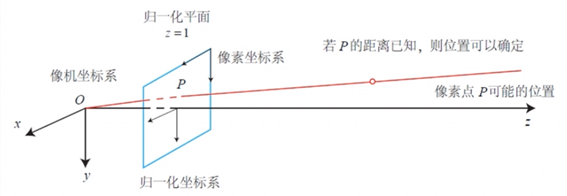主要包括四种坐标：世界坐标、相机坐标、归一化相机坐标和像素坐标。 其中O-x-y-z为相机坐标系，O为相机的光心，一般z轴指向相机前方，x轴向右，y轴向下；当z=1时称为归一化平面；像素坐标系定义为：原点O‘位于图像左上角，u轴向右与x轴平行，v轴向下与y轴平行。
内参的推导： 根据针孔相机的前投影模型得到三维点和成像平面上点的关系：

Z

f

=

X

X

′

=

Y

Y

′

⇒

X

′

=

f

X

Z

，

Y

′

=

f

Y

Z

\frac{Z}{f}=\frac{X}{X&#x27;}=\frac{Y}{Y&#x27;}\Rightarrow X&#x27;=f\frac{X}{Z}，Y&#x27;=f\frac{Y}{Z}

再从成像平面到像素坐标：

u

=

α

X

′

+

c

x

，

v

=

β

Y

′

+

c

y

u=\alpha X&#x27;+c_x，v=\beta Y&#x27;+c_y

整理得到：

[

u

v

1

]

=

1

Z

[

f

x

0

c

x

0

f

y

c

y

0

0

1

]

[

X

Y

Z

]

≜

1

Z

K

P

\begin{bmatrix}u\\v\\1 \end{bmatrix}=\frac{1}{Z}\begin{bmatrix}fx&amp;0&amp;c_x\\0&amp;fy&amp;c_y\\0&amp;0&amp;1 \end{bmatrix}\begin{bmatrix}X\\Y\\Z \end{bmatrix} \triangleq \frac{1}{Z}KP

针孔模型总体流程：
1、世界坐标系下有一个点P，世界坐标为：

P

w

P_w

2、相机的运动由R、t描述，将其转移到相机坐标系下为：

P

ˉ

c

=

R

P

w

+

t

\bar P_c=RP_w+t

3、将相机坐标投影到归一化平面上，得到归一化相机坐标：

P

c

=

[

X

/

Z

,

Y

/

Z

,

1

]

P_c = [X/Z,Y/Z,1]

4、归一化坐标经过内参后对应到其像素坐标：

P

u

v

=

K

P

c

P_{uv}=KP_c

1.2 相机畸变
为了获得更好的成像效果，会在相机前方安装滤镜。滤镜自身的形状对光纤传播产生影响，引起的畸变称为径向畸变，主要分为桶形畸变和枕型畸变；在机械组装过程中不能保证透镜和成像平面严格平行产生的时切向畸变。
对于归一化平面上一点

p

=

[

x

,

y

]

T

p=[x,y]^T

，也可以写成极坐标的形式

[

r

,

θ

]

T

[r,\theta]^T

，假设畸变后点的坐标为

[

x

d

i

s

t

o

r

t

e

d

,

y

d

i

s

t

o

r

t

e

d

]

[x_{distorted},y_{distorted}]

。对于径向畸变有：

x

d

i

s

t

o

r

t

e

d

=

x

(

1

+

k

1

r

2

+

k

2

r

4

+

k

3

r

6

)

x_{distorted}=x(1+k_1r^2+k_2r^4+k_3r^6)

y

d

i

s

t

o

r

t

e

d

=

y

(

1

+

k

1

r

2

+

k

2

r

4

+

k

3

r

6

)

y_{distorted}=y(1+k_1r^2+k_2r^4+k_3r^6)

对于切向畸变有：

x

d

i

s

t

o

r

t

e

d

=

x

+

2

p

1

x

y

+

p

2

(

r

2

+

2

x

2

)

x_{distorted}=x+2p_1xy+p_2(r^2+2x^2)

y

d

i

s

t

o

r

t

e

d

=

y

+

p

1

(

r

2

+

2

y

2

)

+

2

p

2

x

y

y_{distorted}=y+p_1(r^2+2y^2)+2p_2xy

去畸变流程：对于每个归一化坐标

(

x

,

y

)

(x,y)

，找到其在原始（畸变）图像上的坐标

(

x

d

i

s

t

o

r

t

e

d

,

y

d

i

s

t

o

r

t

e

d

)

(x_{distorted},y_{distorted})

，然后将纠正后的点通过内参数投影到像素平面，得到该点在图像上的正确位置。
1.3 相机标定（张正友标定）
相机标定的主要目的是确定相机的内参K与畸变系数，在标定过程中也会求得相机的运动即外参。
这里主要介绍最常用的张氏标定，即棋盘格标定，其是介于传统标定方法和自标定方法之间的一种方法。注意张氏标定仅考虑了径向畸变而没有切向畸变。
1）求单应性矩阵H 设棋盘格中一点的三维世界坐标

P

=

[

X

,

Y

,

Z

,

1

]

T

P=[X,Y,Z,1]^T

，像素坐标为

m

=

[

u

,

v

,

1

]

T

m=[u,v,1]^T

，得棋盘格平面到图像平面的单应性关系为：

s

m

=

K

[

R

,

T

]

X

sm=K[R,T]X

令相机内参K为：

[

α

γ

u

0

0

β

v

0

0

0

1

]

\begin{bmatrix}\alpha &amp; \gamma &amp; u_0\\0 &amp; \beta &amp; v_0\\0&amp;0&amp;1 \end{bmatrix}

令棋盘格所在平面为Z=0平面，可得：

s

[

u

v

1

]

=

K

[

r

1

r

2

r

3

t

]

[

X

Y

0

1

]

=

K

[

r

1

r

2

t

]

[

X

Y

1

]

s\begin{bmatrix}u\\v\\1 \end{bmatrix}=K\begin{bmatrix}r_1 &amp; r_2 &amp; r_3 &amp;t \end{bmatrix}\begin{bmatrix}X\\Y\\0\\1 \end{bmatrix}=K\begin{bmatrix}r_1 &amp; r_2 &amp;t \end{bmatrix}\begin{bmatrix}X\\Y\\1 \end{bmatrix}

[

u

v

1

]

=

1

s

K

[

r

1

r

2

t

]

[

X

Y

1

]

≜

H

[

X

Y

1

]

\begin{bmatrix}u\\v\\1 \end{bmatrix}=\frac{1}{s} K\begin{bmatrix}r_1 &amp; r_2 &amp;t \end{bmatrix}\begin{bmatrix}X\\Y\\1 \end{bmatrix} \triangleq H\begin{bmatrix}X\\Y\\1 \end{bmatrix}

得到两个平面的单应性矩阵

H

=

[

h

1

,

h

2

,

h

3

]

H=[h_1,h_2,h_3]

，其是一个齐次方程，有8个未知数，每对对应点提供两个方程，所以需要4对对应点可以算出世界平面到图像平面的单应性矩阵H。
2）求内参矩阵 由上述得

r

1

=

s

K

−

1

h

1

，

r

2

=

s

K

−

1

h

2

r_1 = sK^{-1}h_1，r_2 = sK^{-1}h_2

由于旋转矩阵正交性，可得：

r

1

T

r

2

=

0

，

∣

∣

r

1

∣

∣

=

∣

∣

r

2

∣

∣

=

1

r_1^Tr_2=0，||r_1||=||r_2||=1

代入有：

h

1

T

K

−

T

K

−

1

h

2

=

0

，

h

1

T

K

−

T

K

−

1

h

1

=

h

2

T

K

−

T

K

−

1

h

2

h_1^TK^{-T}K^{-1}h_2=0，h_1^TK^{-T}K^{-1}h_1=h_2^TK^{-T}K^{-1}h_2

每个单应性矩阵提供两个方程，而内参矩阵包含5个参数，至少需要3个单应性矩阵
3）计算外参矩阵 根据

1

s

K

[

r

1

r

2

t

]

=

H

=

[

h

1

h

2

h

3

]

\frac{1}{s} K\begin{bmatrix}r_1 &amp; r_2 &amp;t \end{bmatrix}= H = \begin{bmatrix}h_1 &amp;h_2 &amp;h_3\end{bmatrix}

，我们已经得到了H和K，可得：

s

=

1

∣

∣

K

−

1

h

1

∣

∣

=

1

∣

∣

K

−

1

h

2

∣

∣

s=\frac{1}{||K^{-1}h_1||}=\frac{1}{||K^{-1}h_2||}

r

1

=

s

K

−

1

h

1

，

r

2

=

s

K

−

1

h

2

r_1 = sK^{-1}h_1，r_2 = sK^{-1}h_2

r

3

=

r

1

×

r

2

r_3 = r_1\times r_2

t

=

s

K

−

1

h

3

t = sK^{-1}h_3

4）极大似然估计 上述推导了理论值，然而实际测量存在高斯噪声，因此需要使用最大似然估计进行优化，假设采集了n副棋盘格图像，每个棋盘格上检测到角点m个，其中第i副棋盘格上的角点Mj的像素投影点为：

m

^

(

K

,

R

i

,

t

i

,

M

i

j

)

=

K

[

R

∣

t

]

M

i

j

\hat m(K,R_i,t_i,M_{ij})=K[R|t]M_{ij}

构造似然函数并使用LM算法迭代求解非线性最小二乘问题，并采用之前计算的理论值作为初值：

∑

i

=

1

n

∑

j

=

1

m

∣

∣

m

i

j

−

m

^

(

K

,

R

i

,

t

i

,

M

i

j

)

∣

∣

2

\sum^n_{i=1}\sum^m_{j=1} ||m_{ij}-\hat m(K,R_i,t_i,M_{ij})||^2

5）径向畸变估计 张氏标定只关注影响最大的径向畸变。根据之前的径向畸变方程：

u

d

i

s

t

o

r

t

e

d

=

u

+

(

u

−

u

0

)

(

k

1

r

2

+

k

2

r

4

)

u_{distorted}=u+(u-u_0)(k_1r^2+k_2r^4)

v

d

i

s

t

o

r

t

e

d

=

v

+

(

v

−

v

0

)

(

k

1

r

2

+

k

2

r

4

)

v_{distorted}=v+(v-v_0)(k_1r^2+k_2r^4)

化成矩阵形式：

[

(

u

−

u

0

)

r

2

(

u

−

u

0

)

r

4

(

v

−

v

0

)

r

2

(

v

−

v

0

)

r

4

]

[

k

1

k

2

]

=

[

u

d

i

s

t

o

r

t

e

d

−

u

v

d

i

s

t

o

r

t

e

d

−

v

]

\begin{bmatrix}(u-u_0)r^2 &amp;(u-u_0)r^4\\(v-v_0)r^2&amp;(v-v_0)r^4 \end{bmatrix}\begin{bmatrix}k_1\\k_2 \end{bmatrix}=\begin{bmatrix}u_{distorted}-u\\v_{distorted}-v \end{bmatrix}

记为：

D

k

=

d

⇒

k

=

(

D

T

D

)

−

1

D

T

d

Dk=d\Rightarrow k=(D^TD)^{-1}D^Td

，然后再对最大似然估计进行优化。
1.4 全局快门（global shutter）和卷帘快门（rolling shutter）
全局快门是在同一时间进行曝光，其曝光时间短，会增加噪点；卷帘快门时通过传感器逐行扫描进行曝光，不同行像元的曝光时间不同，因此当曝光不当或物体移动较快时，会出现部分曝光(partial exposure)、斜坡图形(skew)、晃动(wobble) 等现象（称为果冻效应），但是可以达到更高的帧数和更低的噪声。

2、立体相机模型
2.1 双目相机模型
双目相机一般由两个水平放置的相机组成，可以将其看作针孔相机模型。两者的距离称为刷双目相机的基线。
假设空间点P在左右相机各成的像为

P

L

、

P

R

P_L、P_R

，理想情况下两者只在图像x轴上有差异，记左侧坐标为

u

L

u_L

，右侧坐标为

u

R

u_R

，根据三角形相似关系有：

z

−

f

z

=

b

−

u

L

+

u

R

b

\frac{z-f}{z}=\frac{b-u_L+u_R}{b}

可得：

z

=

f

b

d

，

d

=

u

L

−

u

R

z=\frac{fb}{d}，d=u_L-u_R

这里d为左右两图的横坐标之差，称为视差。由于视差最小为一个像素，双目深度存在一个理论上的最大值，由

f

b

fb

决定。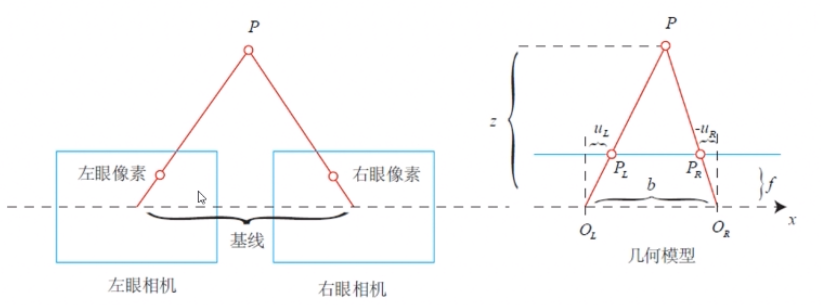2.2 RGBD相机原理
这部分想详细了解推荐看博客 https://blog.csdn.net/electech6/article/details/78707839 和 https://blog.csdn.net/electech6/article/details/78349107
RGBD相机原理主要分为： 1、通过红外结构光(Structured Light)来测量像素距离，如Kinect1、Project Tango1、Intel Realsense等； 通过近红外激光器，将具有一定结构特征的光（比如离散光斑、条纹光、编码结构光等）投射到物体上，再由专门的红外摄像头进行采集。然后通过返回的结构光图案，计算物体与自身的距离。
2、通过飞行时间法（TOF）原理来测量像素距离，如Kinect2、TOF传感器等。 通过发射脉冲光到被观测物体上，然后接收从物体反射回去的脉冲光，通过发送到返回的光束飞行（往返）时间来计算被测物体离相机的距离。分为光脉冲调制和连续波调制。
2.3 双目相机与RGBD相机对比
（部分数据可能是前几年的技术水平，仅供参考，但局限性与基本趋势不会变）
相机TOF结构光双目测距方式主动式主动式被动式工作原理根据红外(IR)光飞行时间直接测量主动投影已知编码图案图像特征点匹配，三角测量间接计算帧率较高，可达上百FPS30fps从高到低都有（一般较低）分辨率低于640X480可达1080X720可达2K测量精度最高里厘米近距离可达0.01-1mm近距离可达毫米级测量范围可测量较远距离一般10m以内由于基线限制，只能测量较近距离强光环境中弱良好低光环境良好良好弱影响因素不受光照变化和物体纹理影响，受多重反射影响容易受光照影响，难以用于室外受光照变化和物理纹理影响很大，夜晚无法使用硬件成本高中低软件复杂度低中高功耗很高，需全面照射中，只需照射局部区域低
展开全文双目相机 RGBD 结构光
• 一 理论基础 关于针孔相机模型，参考博客； 关于鱼眼相机模型，参考参考文献。 这里只需要知道我们这里使用的鱼眼相机模型是等距投影的鱼眼相机模型，即r=fθ(1)，而针孔相机模型是透视投影，即r=ftanθ(2)...
一 理论基础
关于针孔相机模型，参考博客；
关于鱼眼相机模型，参考参考文献。
这里只需要知道我们这里使用的鱼眼相机模型是等距投影的鱼眼相机模型，即r=fθ(1)，而针孔相机模型是透视投影，即r=ftanθ(2)。其中f是焦距focus，r是成像平面内某像素点到主光点principal point的距离，principal point是成像平面和主光轴的焦点，θ是入射光线和主光轴的夹角。根据(1)和(2)，可以得到从正常图像上某点P(xc,yc)到鱼眼相机上某点P(xf,yf)的映射关系:
rf = f*arctan(rc/f)
二 OpenCV实现
#include <opencv2/core/core.hpp>
#include <opencv2/highgui/highgui.hpp>
#include <iostream>
#include <cmath>

int main() {
cv::Mat srcImg;
if (srcImg.data != nullptr) {
std::cout << "Load Image Successfully" << std::endl;
}
const int f = 200;
std::cout << srcImg.type() << std::endl;
cv::Mat dstImg = cv::Mat(srcImg.cols, srcImg.cols, CV_8UC3, cv::Scalar(0,0,0));
//---------transform pinhole image to fisheye image---------------//
int ux = srcImg.cols / 2;
int uy = srcImg.rows / 2;
std::cout << uy << std::endl;
int dx, dy, xf, yf, x, y;
double rc, rf, theta, gama;
for (int i = 0; i < srcImg.rows; i++) {
for (int j = 0; j < srcImg.cols; j++) {
dy = i - uy;
dx = j - ux;
rc = sqrt(pow(dy, 2) + pow(dx, 2));
theta = atan2(rc, f);
gama = atan2(dy, dx);
rf = f * theta;
xf = rf * cos(gama);
yf = rf * sin(gama);
x = xf + ux;
y = yf + uy;
dstImg.at<cv::Vec3b>(y, x) = srcImg.at<cv::Vec3b>(i, j);
dstImg.at<cv::Vec3b>(y, x) = srcImg.at<cv::Vec3b>(i, j);
dstImg.at<cv::Vec3b>(y, x) = srcImg.at<cv::Vec3b>(i, j);
}
}
//----------------------------------------------------------------//
cv::namedWindow("srcImg");
cv::namedWindow("dstImg");
cv::imshow("srcImg", srcImg);
cv::imshow("dstImg", dstImg);
cv::waitKey(0);
cv::destroyAllWindows();
return 0;
}
效果如下：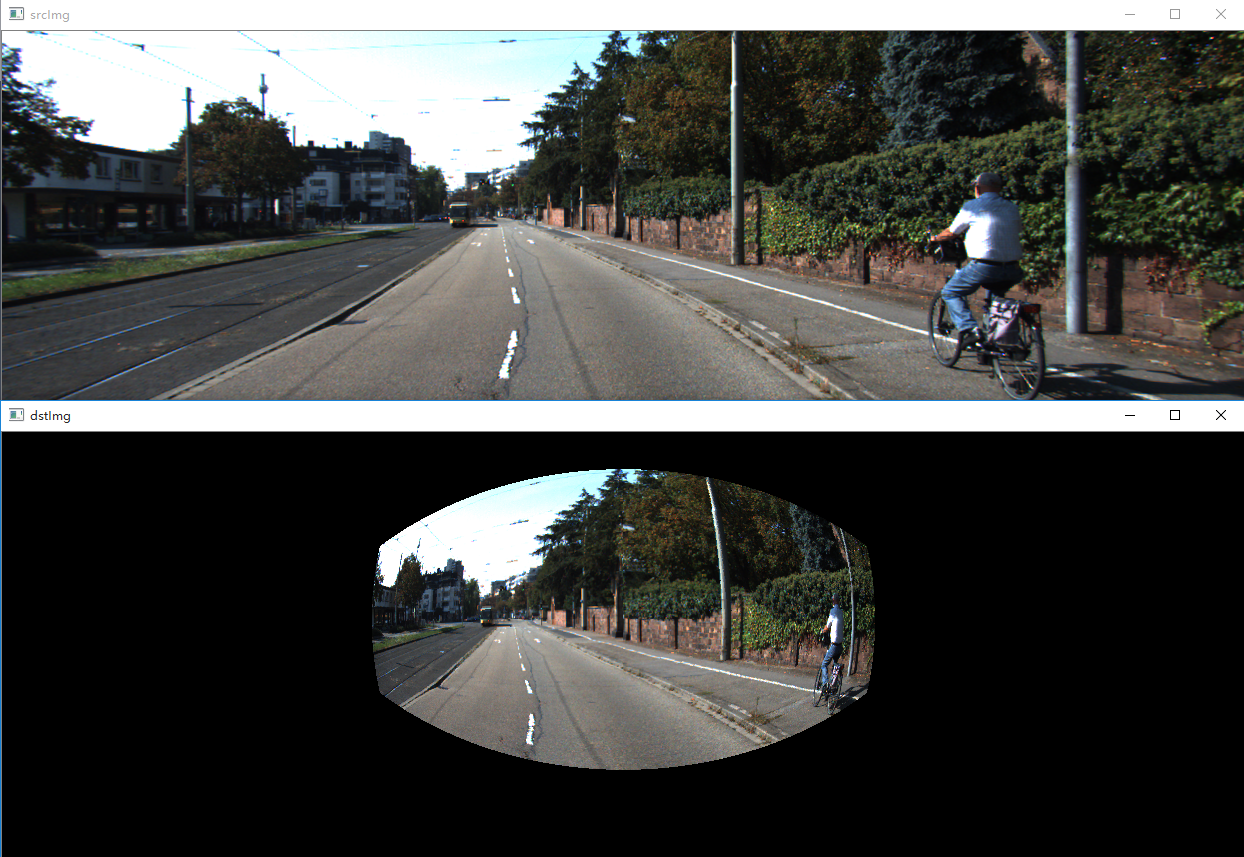三 代码实现过程总结
1 求取dy的平方时，用pow()函数，dy ^ 2表示dy和2进行位异或操作，还是码得少啊...
2 用一张像素值为0的图片初始化dstImg，cv::Mat dstImg = cv::Mat(rows, cols, CV_8UC3, cv::Scalar(0,0,0));

参考文献：
K. Miyamoto, "Fish Eye Lens," Journal of the Optical Society of America, vol. 54, 1964.
J. Kannala and S. S. Brandt, "A generic camera model and calibration method for conventional, wide-angle, and fish-eye lenses," IEEE Transactions on Pattern Analysis & Machine Intelligence, vol. 28, pp. 1335-40, 2006.
展开全文opencv
• ## 相机模型详解

千次阅读 2018-05-09 15:39:23
相机模型数码相机图像拍摄的过程实际上是一个光学成像的过程。相机的成像过程涉及到四个坐标系：世界坐标系、相机坐标系、图像坐标系、像素坐标系以及这四个坐标系的转换。理想透视模型——针孔成像模型相机模型是...
相机模型
数码相机图像拍摄的过程实际上是一个光学成像的过程。相机的成像过程涉及到四个坐标系：世界坐标系、相机坐标系、图像坐标系、像素坐标系以及这四个坐标系的转换。
理想透视模型——针孔成像模型
相机模型是光学成像模型的简化，目前有线性模型和非线性模型两种。实际的成像系统是透镜成像的非线性模型。最基本的透镜成像原理如图所示：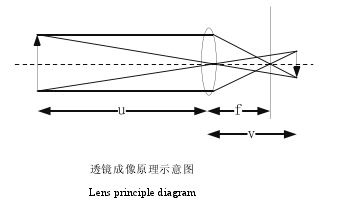其中 u 为物距， f 为焦距，v 为相距。三者满足关系式：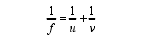相机的镜头是一组透镜，当平行于主光轴的光线穿过透镜时，会聚到一点上，这个点叫做焦点，焦点到透镜中心的距离叫做焦距 f。数码相机的镜头相当于一个凸透镜，感光元件就处在这个凸透镜的焦点附近，将焦距近似为凸透镜中心到感光元件的距离时就成为小孔成像模型。小孔成像模型如图所示。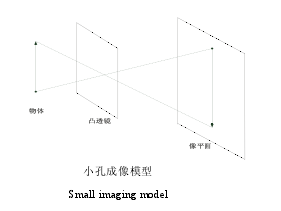小孔成像模型是相机成像采用最多的模型。在此模型下，物体的空间坐标和图像坐标之间是线性的关系，因而对相机参数的求解就归结到求解线性方程组上。四个坐标系的关系图如下图所示，其中 M 为三维空间点，m 为 M 在图像平面投影成的像点。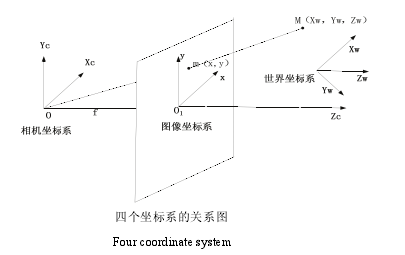①世界坐标系：是客观三维世界的绝对坐标系，也称客观坐标系。因为数码相机安放在三维空间中，我们需要世界坐标系这个基准坐标系来描述数码相机的位置，并且用它来描述安放在此三维环境中的其它任何物体的位置，用（Xw, Yw, Zw）表示其坐标值。
②相机坐标系（光心坐标系）：以相机的光心为坐标原点，X 轴和Y 轴分别平行于图像坐标系的 X 轴和Y 轴，相机的光轴为Z 轴，用（Xc, Yc, Zc）表示其坐标值。
③图像坐标系：以CCD 图像平面的中心为坐标原点，X轴和Y 轴分别平行于图像平面的两条垂直边，用( x , y )表示其坐标值。图像坐标系是用物理单位（例如毫米）表示像素在图像中的位置。
④像素坐标系：以 CCD 图像平面的左上角顶点为原点，X 轴和Y 轴分别平行于图像坐标系的 X 轴和Y 轴，用(u , v )表示其坐标值。数码相机采集的图像首先是形成标准电信号的形式，然后再通过模数转换变换为数字图像。每幅图像的存储形式是M × N的数组，M 行 N 列的图像中的每一个元素的数值代表的是图像点的灰度。这样的每个元素叫像素，像素坐标系就是以像素为单位的图像坐标系。
像素坐标系与图像坐标系的关系如图。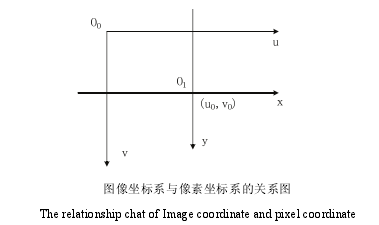他们之间的转换关系为：采用齐次坐标再用矩阵形式将上式表示为：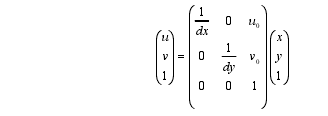其中（u0, v0）是图像坐标系原点在像素坐标系中的坐标，dx 和 dy分别是每个像素在图像平面x和 y方向上的物理尺寸。
图像坐标系与相机坐标系的转换为：其中 f 为焦距（像平面与相机坐标系原点的距离）。用齐次坐标系和矩阵表示上述关系：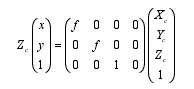相机坐标系与世界坐标系的变换为：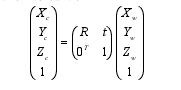其中 R 为3 × 3正交旋转矩阵，t 为三维平移向量，综合起来：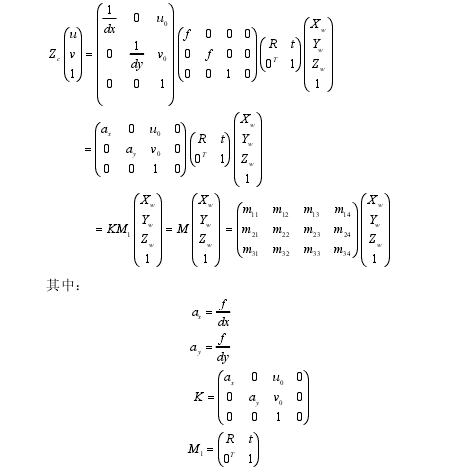ax, ay分别是图像水平轴和垂直轴的尺度因子。K的参数中只包含焦距、主点坐标等只由相机的内部结构决定，因此称 K 为内部参数矩阵，ax, ay , u0, v0叫做内部参数。M1中包含的旋转矩阵和平移向量是由相机坐标系相对于世界坐标系的位置决定的，因此称M1为相机的外部参数矩阵，R和t叫做外部参数，M 叫投影矩阵。相机标定就是确定相机的内部参数和外部参数。
实际成像模型
理想的透视模型是针孔成像模型，物和像会满足相似三角形的关系。但是实际上由于相机光学系统存在加工和装配的误差，透镜就并不能满足物和像成相似三角形的关系，所以相机图像平面上实际所成的像与理想成像之间会存在畸变。畸变属于成像的几何失真，是由于焦平面上不同区域对图像的放大率不同形成的画面扭曲变形的现象，这种变形的程度从画面中心至画面边缘依次递增，主要在画面边缘反映比较明显。为了减小畸变，拍摄图片时应尽量避免用镜头焦距的最广角端或最远端拍摄。实际的相机成像模型如下图所示。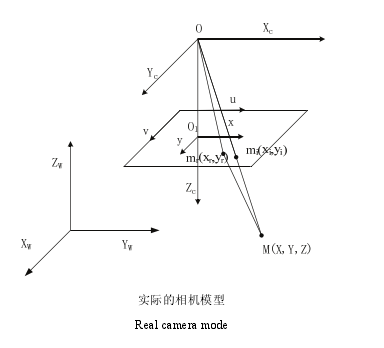其中 mr(xr,yr)表示实际投影点的像平面坐标系下的物理坐标，mi(xi,yi)表示理想投影点的像平面坐标系下的物理坐标。镜头的畸变模型可表示为：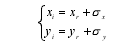σx 和σy是非线性畸变值，它包括径向畸变和偏心畸变和薄棱镜畸变等。理论上来说镜头都存在径向和切向畸变，但是通常径向畸变较大，切向畸变较小。径向畸变的模型可由下面的模型来表示：其中k1 k2 k3……示径向畸变系数，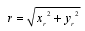，通常情况下径向畸变系数只考虑到一阶或二阶就可以满足精度需求了。
偏心畸变模型是由于多个光学镜头的光轴不能完全共线产生的，这种畸变是由径向和切向畸变共同构成的，数学模型可表示如下：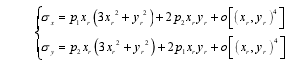其中p1, p2为切向畸变系数。薄棱镜畸变是由于镜头设计制造缺陷和加工安装所造成的，如镜头与相机成像平面有一个很小的倾角等。因为薄棱镜畸变非常小，通常不考虑，这里只考虑径向畸变和偏心畸变，畸变总的可以表示为：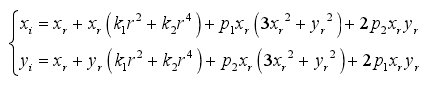实际的成像模型为：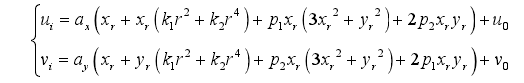******************
作者：hao_09
时间：2015/8/13
文章地址：http://blog.csdn.net/lsh_2013/article/details/47615309
******************
展开全文机器视觉
• 本文提出了一种具有单个有效视点约束的球面相机模型，用于发散相机集群。我们将球面投影定义为使用中心投影在球面上表示空间点的方法。因此，将发散的相机群集视为解决计算机视觉问题的单个模型是合理的。为了构建...
• 转载，标记：相机模型，内参的详细推到 相机标定原理介绍（一）
•研究论文
• ## 鱼眼相机模型

千次阅读 2018-03-06 16:09:17
原文地址：http://blog.sciencenet.cn/blog-465130-1052526.html理想相机模型的映射函数：对于单目相机而言，空间的3D点的成像过程可以表达为空间点P(物点)与光心O的连线在成像面上的投影p(像点)，机器视觉中...
• 本文首先介绍SLAM中常用的相机模型和畸变模型，随后介绍我们采用的两种内参标定方案：chessboard和Apriltag。 1.1相机模型和畸变模型 这里主要介绍pinhole模型和fisheye模型，简单涉猎omnidirection模型. 成...
• 相机模型 数码相机图像拍摄的过程实际上是一个光学成像的过程。相机的成像过程涉及到四个坐标系：世界坐标系、相机坐标系、图像坐标系、像素坐标系以及这四个坐标系的转换。 理想透视模型——针孔成像模型 ...
• ## 相机模型(一)

千次阅读 2019-05-27 17:10:36
相机模型相机与图像一些基本概念针孔相机模型坐标系描述成像过程1、世界坐标系->相机坐标系2、相机坐标系-->象平面坐标系3、象平面坐标系-->像素平面坐标系4、世界坐标到像素坐标畸变 相机与图像 一些基本...立体视觉 小孔成像
• ## 相机模型公式推导

千次阅读 2019-03-08 16:13:40
相机模型，主要有finite camera和infinite camera。 其中finite camera就是我们常说的针孔模型，本文对针孔模型的成像过程进行了推导。推导过程如下： (a)先假设相机坐标系与世界坐标系重合，推导从相机坐标系到...计算机视觉
• 针孔相机、鱼眼相机模型 一、针孔相机模型 空间的三维物体要成像到相机的 CMOS/CCD 上面，形成了图像。图像上的每个点对应空间上的一个点。 将世界坐标系上的一点 (x,y,z)映射到CMOS/CCD图像坐标系上，只要两步： ...计算机视觉
• ## 相机模型成像原理

千次阅读 2018-07-14 10:19:31
本内容为slam学习中所涉及的关于相机模型与非线性优化的基础知识整理。一.针孔相机模型与图像照片记录了真实世界在成像平面上的投影，这个过程丢弃了“距离”维度上的信息，普通相机可以用针孔模型很好的近似。小孔...
• 针对这一问题,提出一种完整的聚焦型光场相机等效多目相机模型。在此基础上,利用传统多目相机的SFM算法,给出了适用于聚焦型光场相机的位姿估计算法示例和点云三角化算法示例。最后,通过仿真实验和真实场景重建实验...
• 代码解读-VINS_Mono中的鱼眼相机模型，内含自动驾驶完整学习资料
• 想到学习下相机模型，是因为最近做的事情涉及到求解相机参数、恢复相机位置等。而在这里开博，主要也是当做学习笔记来用。 在相机模型中，针孔相机是相对简单而常用的模型。由于其用的是投影成像的方法，因此要先...计算机视觉
• 针孔相机模型 相机坐标转换成像素坐标，由内参数矩阵决定。 相机位姿决定世界坐标转换成相机坐标：即外参数矩阵 内参数矩阵K为3X3矩阵，对于三维空间点，旋转矩阵R和平移矩阵t构成的外参数矩阵T为4X4矩阵，为了...
• Omnidirectional Camera Davide Scaramuzza Definition 定义 omnidirectional camera (from omni, meaning all) 广角相机： 在一个水平面有360度...大多数商业相机可以使用 pinhole 相机模型来描述，使用一个 per...
• RPCM-有理多项式相机模型 用于光学卫星图像的有理多项式相机 (RPC) 模型的 Python 实现。 , Gabriele Facciolo, Enric Meinhardt-Llopis (CMLA, ENS Cachan, Université Paris-Saclay) 2013-19 rpcm是一个 Python...Python
• Pinhole camera model相机模型计算机视觉 图像处理
• 这节博客介绍一下计算机视觉中的几何问题之一：相机模型和坐标转换，希望对各位读者有所帮助！ 1.针孔相机模型——几何建模 数码相机，手机镜头可以简单看作是一组透镜和图像传感器构成的整个光学成像系统。透镜...
• 相机模型 除进行高精度尺寸测量使用远心镜头外，我们正常使用的相机镜头都是普通镜头，其成像模型都是理想的小孔成像模型。 我们在光轴中心处建立坐标系，Z轴方向平行于光轴，正方向为相机到拍摄对象的方向，X...
• 鱼眼相机模型模型介绍等距投影等立体角投影正交投影体视投影线性投影 之前总结了一下针孔相机的模型，然后得到了比较积极的回复（其实是我到处求人关注的，虽然截至到目前才三个人），所以就再接再励，乘胜追击（也...
• 神经网络和自适应遗传退火算法校准的非线性相机模型研究论文...